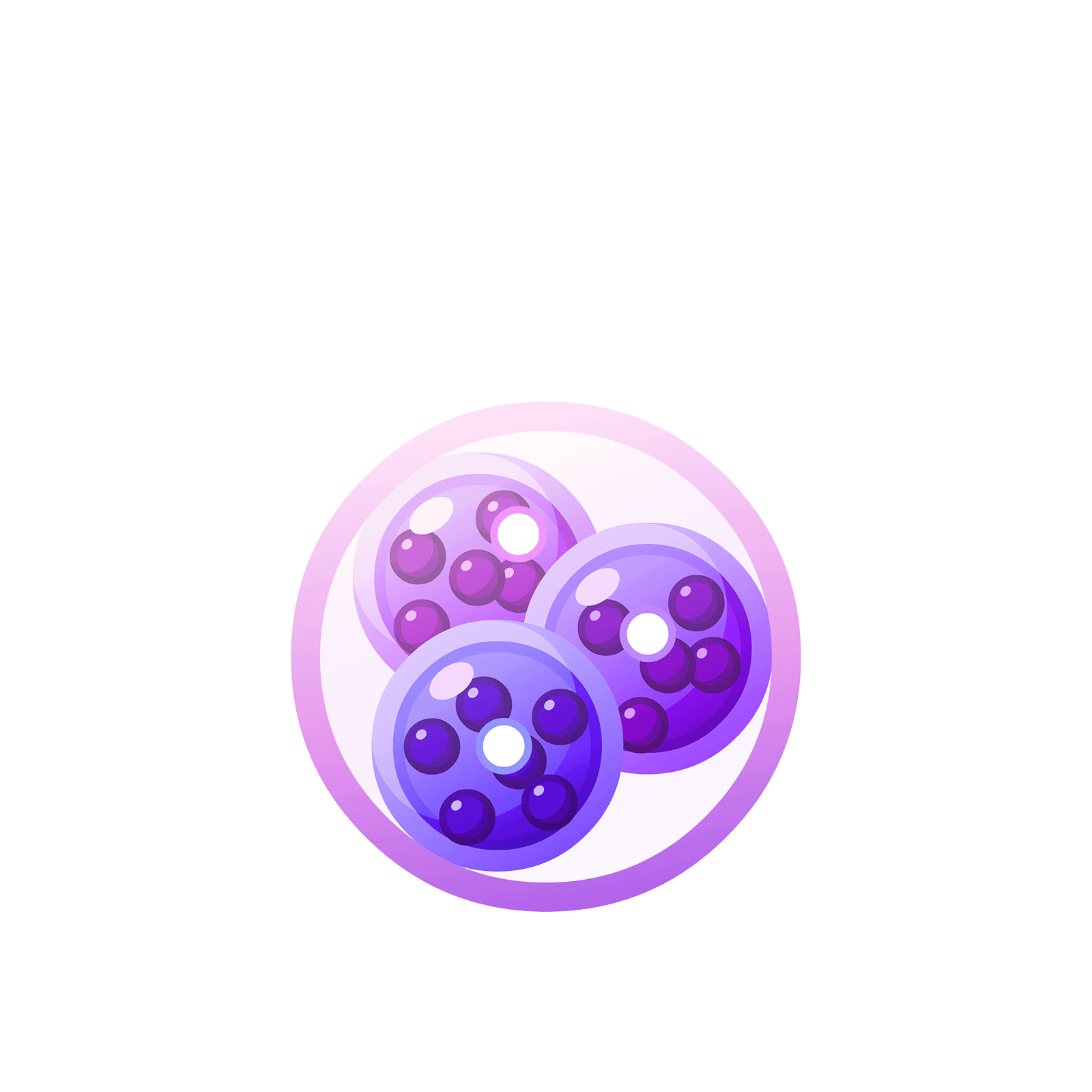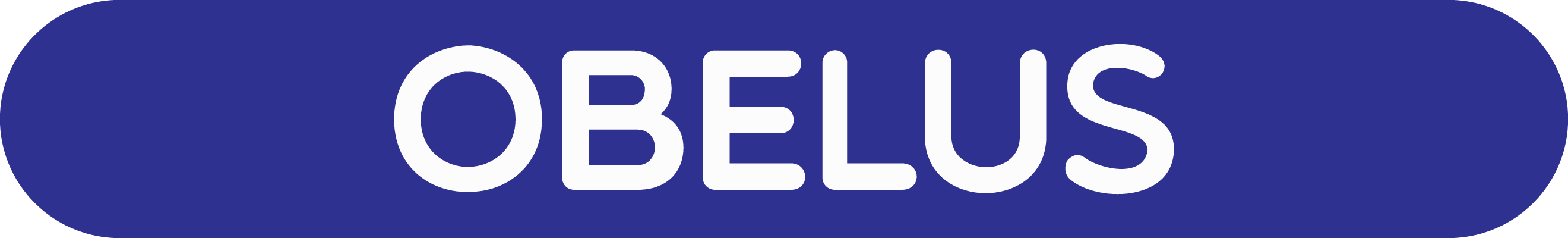05 217 385# Station  MFurther ExplanationAs one mulls on the long-division process you come to realize that there are subtle issues at play. (Maybe that is why you came to this station on the island?)

### Question 1

Let’s take some time here to think through division more slowly. And let’s start with the example whose answer we can write down right away.

What is $3906 \div 3$?

Answer: $1302$.

What makes us able to see this answer so swiftly? It seems natural to think of $3906$ as $3000 + 900 + 6$. It is easy to divide each of these components by three.

Dividing
$3906 = 3000 + 900 + 6$
by three gives
$3906 \div 3 = 1000 + 300 + 2 = 1302$.

Great! And we see this natural decomposition too in a dots and boxes picture of $3906$. We literally see $3$ thousands, $9$ hundreds, and $6$ ones.Dividing by three gives this picture.But let’s probe even deeper into the workings of this final division step. What really happened here?

We can think of division as a task of grouping. “What is $3906 \div 3 = ?$” is really asking

How many groups of three can you find among a collection of $3906$ objects?

We know that there one thousand groups of three among $3000$ dots, and three-hundred groups of three among $900$ dots, and two groups of three among 6 dots. And our picture of $3906$ actually shows this too.If we did all the unexplosions, the green loop of dots would unexplode to give one-thousand green loops in the ones place. Each pink loop of dots unexplodes to make one-hundred pink loops in the ones place, and as there are three pink loops we get a total of three-hundred pink loops in the ones place… We see that out picture is really one of one-thousand green loops, three-hundred pink loops, and two orange loops. We have $1302$ groups of three.

We can use tally marks to show that we have $1$ group of three at the thousands level, $3$ at the hundreds level, $0$ at the tens level, and $2$
at the ones level, again $1302$ groups of three.And these tally marks show what happens if we were to actually divide by three: each group of three dots becomes one dot. We’d get this picture.This final picture shows how many groups of three we had in the original $3906$. But we don’t actually need to draw this final picture: the tally marks in the picture before it show this information too. So we can stop drawing once we’ve figured out all the tallies.

Try performing the division problem $3906 \div 3$ in the $1 \leftarrow 10$ machine.

Try performing the division problem $3906 \div 3$ in the $1 \leftarrow 10$ machine.### Question 2

Draw a dots and boxes picture of the number $426$ and use it explain why $426 \div 2$ equals $213$.

Try it on the app too.### Question 3

Let’s do another problem.

Let’s try $402 \div 3$.### Question 4

We just showed that $402 \div 3 = 134$.

What do you think is the answer, then, to $404 \div 3$?### Question 5

Compute $61230 \div 5$ by the dots-and-boxes approach. Try it on the app too.
(Does it get tiresome drawing dots? Do you have to actually draw them?)Exactly the same thinking applies to multi-digit division too. In station 11 we looked at $276 \div 12$.

Here’s picture of $276$.Here’s what twelve dots look like.But in a $1 \leftarrow 10$ machine we’d really see them as one dot next to two dots after an explosion. (All twelve dots still really reside in the rightmost box.)And when we hunt for groups of twelve in $276$, we get this picture.We see two groups of $12$ at the tens level and three $12$s at the ones level. The answer to $276 \div 12$ is indeed $23$.

### Let's Go Wild!